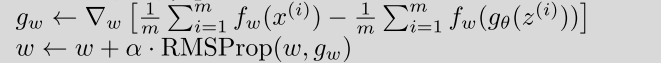# Question of PyTorch

What is the meaning of following PyTorch codes(from wgan lessons)?

``````one = torch.FloatTensor()
D_real = netD(real_data_v) //netD is discriminator network
D_real = D_real.mean() //find the mean
D_real.backward(one)
``````

D_real is a PyTorch variable, usually we do not pass any parameter into the backward function, what is the meaning of passing a float tensor into it?Thanks

1 Like

Hi @tham, in pseudo code provided in the wgan paper, the part where they calculate discriminator/critique loss,D_real is the first term in the first equation.There is a +ve sign to it.D_fake is the second term in it which has -ve sign.
The parameter we pass to backward() will simply multiply gradients with it

It is reasonable if we pass -1 to backward function, but why do we pass 1 into backward function?

Any value * 1 = Any value

I guess it shouldn’t make any difference ?